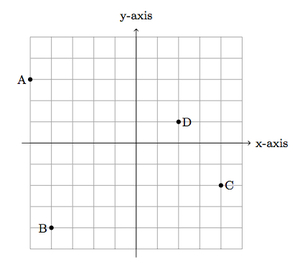# Reflecting points over coordinate axes

Alignments to Content Standards: 6.NS.C.6.b

Below are some points in the coordinate plane:1. Find the coordinates of the points.
2. Reflect the points over the $x$-axis and find the coordinates of the new points: label the reflection of point $A$ as $A^\prime$, the reflection of $B$ as $B^{\prime}$, the reflection of $C$ as $C^\prime$, and the reflection of $D$ as $D^\prime$.
3. Reflect the points from (b) over the $y$-axis: label the reflection of point $A^\prime$ as $A^{\prime \prime}$, the reflection of $B^\prime$ as $B^{\prime \prime}$, the reflection of $C^\prime$ as $C^{\prime \prime}$, and the reflection of $D^\prime$ as $D^{\prime \prime}$.
4. How do the points $A^{\prime \prime}$, $B^{\prime \prime}$, $C^{\prime \prime}$, $D^{\prime \prime}$ from (c) relate to the points $A$, $B$, $C$, and $D$?

## IM Commentary

The goal of this task is to give students practice plotting points and their reflections. The teacher may wish to prompt students to identify patterns in parts (b) and (c):

• When we reflect a point $(p,q)$ over the $x$-axis, the $x$-coordinate remains the same and the $y$- coordinate changes signs so the image is $(p,-q)$.
• Similarly when we reflect a point $(p,q)$ over the $y$-axis the $y$-coordinate stays the same but the $x$-coordinate changes signs so the image is $(-p,q)$.

This means that if we reflect over the $x$-axis and then the $y$-axis then both coordinates will change signs. Later students will learn that this combination of reflections represents a 180 degree rotation about the origin. Thus the knowledge gained in this task will help students when they study transformations in the 8th grade and high school.

In order to help identify patterns in how the coordinates of the points change, the teacher may suggest for students to make a table of the points and their images after reflecting first over the $x$-axis and then over the $y$-axis:

Point First Reflection (over $x$-axis) Second Reflection (over $y$-axis)
A=(-5,3) (-5,-3) (5,-3)
B=(-4,-4) (-4,4) (4,4)
C=(4,-2) (4,2) (-4,2)
D=(2,1) (2,-1) (-2,-1)

## Solution

1. The coordinates of $A$ are $(-5,3)$ since $A$ is five units to the left of intersection of the axes and  3 units up. Similarly the coordinates of $B$ are $(-4,-4)$ while $C = (4,-2)$ and $D = (2,1)$.

2. Below is a picture of the reflection of each of the four points over the $x$-axis:We have $A = (-5,3)$. Reflecting over the $x$-axis does not change the $x$-coordinate but changes the sign of the $y$-coordinate. So $A^\prime = (-5,-3)$. If we were to fold the plane along the $x$-axis, the points A and A$^\prime$ match up with one another. The same calculations work for the other points: in each case, the $x$-coordinate does not change and the $y$-coordinate changes sign.

3. Below is a picture of the original points, their reflections over the $x$-axis and then the reflections of the new points over the $y$-axis:Looking again at A$^\prime$ and A$^{\prime \prime}$, we have A$^\prime$ = (-5,-3) and A$^{\prime \prime}$ = (5,-3). Reflecting over the $y$-axis does not change the $y$-coordinate but it changes the sign of the $x$-coordinate.

4. The reflection over the $x$-axis changes the sign of the $y$-coordinate and the reflection over the $x$-axis changes sign of the $y$-coordinate. So the coordinates of P$^{\prime \prime}$ are opposite those of P. For example, A = (-5,3) and A$^{\prime \prime}$ = (5,-3). If we draw a line segment between A and A$^{\prime \prime}$ its midpoint lies at the origin (0,0), and the same is true for all other points.